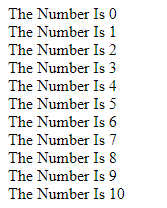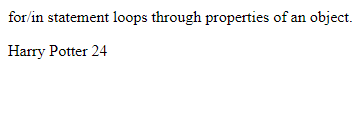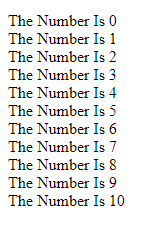# For Loop – JavaScript Basics

With help of Loop a block of code can carry work number of times. Instead of writing the same codes again and again use loop to make your work easy.

Types Of Loops

1. for = use to loop block of code number of times
2. for/in = use to loop properties of an object
3. while = use to loop block of code while stated condition is true
4. do/while = use to loop block of code while stated condition is true

For Loop

```<!DOCTYPE html>
<html>
<body>
<p id="ex"></p>

<script>
var text = "";
var c;
for (c = 0; c < 11; c++) {
text += "The Number Is " + c + "<br>";
}
document.getElementById("ex").innerHTML = text;
</script>

</body>
</html>```

Output :For/In Loop

```<!DOCTYPE html>
<html>
<body>
<p>for/in statement loops through properties of an object.</p>
<p id="ex"></p>

<script>
var txt = "";
var person = {fname:"Harry", lname:"Potter", age:24};
var x;
for (x in person) {
txt += person[x] + " ";
}
document.getElementById("ex").innerHTML = txt;
</script>

</body>
</html>```

Output :While Loop

```<!DOCTYPE html>
<html>
<body>
<p id="ex"></p>

<script>
var text = "";
var a = 0;
while (a < 11) {
text += "<br>The Number Is " + a;
a++;
}
document.getElementById("ex").innerHTML = text;
</script>

</body>
</html>```

Output :Do/While Loop

```<!DOCTYPE html>
<html>
<body>
<p id="ex"></p>
<script>
var text = ""
var a = 0;

do {
text += "<br>The Number Is " + a;
a++;
}
while (a < 11);

document.getElementById("ex").innerHTML = text;
</script>

</body>
</html>```

Output :